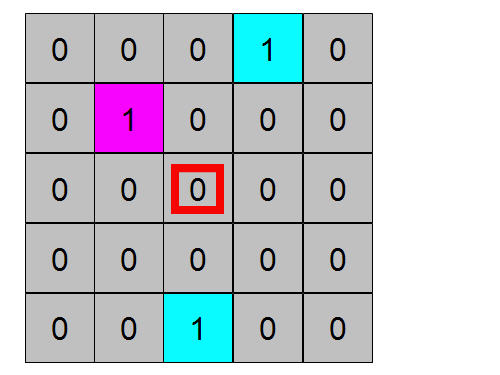# Puzzler: Cleverness needed

I have been working on a side project and I found I needed the following algorithm:Given a binary five by five matrix, I need to find the ‘1’ value that is in the position that has the lowest value in the spiral map above.  I call that the target square. Once that target is found, then I need to return a delta x and delta y that will get me closer to that  target.  Delta x and delta y must be taken relative to the center square, and can only be from the set [-1, 0, 1].

As an example, given the matrix:The ‘1’ in the (2,2) position in the matrix is the target because it maps to 9, and the other two ‘1’ values map to larger values, 11 and 18.  From here, the delta x is -1 and delta y is 1.

If the target was 18, then the values would have been delta x of 0 and delta y of -1.

A final edge case, if the input matrix were all zeros, then delta x and delta y should be chosen randomly, but still pulled from the set [-1, 0, 1].

This is not a particularly difficult algorithm to program if you use 25 if, elseif statements. However, I am looking to be a bit more clever with this.  Please post your solution in the comments, make it a function of this form:

[deltaX, deltaY] = puzzler(inMatrix)

As always with these puzzlers, be sure to use the proper tags in the comments.


<pre> <code>
[deltaX, deltaY] = puzzler(inMatrix)
%% All the code so someone can just copy and paste it from the comments.
</code> </pre>

|

### 댓글

댓글을 남기려면 링크 를 클릭하여 MathWorks 계정에 로그인하거나 계정을 새로 만드십시오.